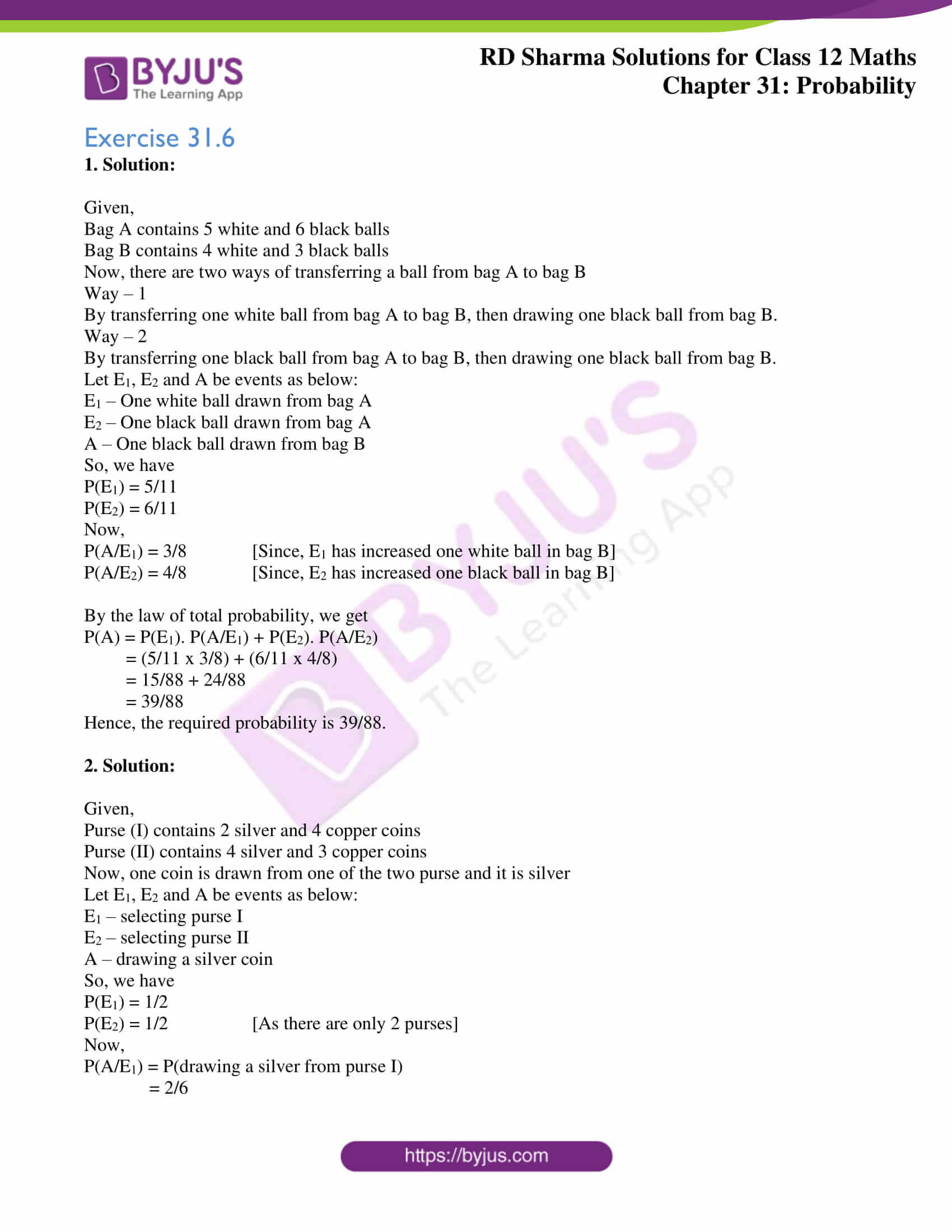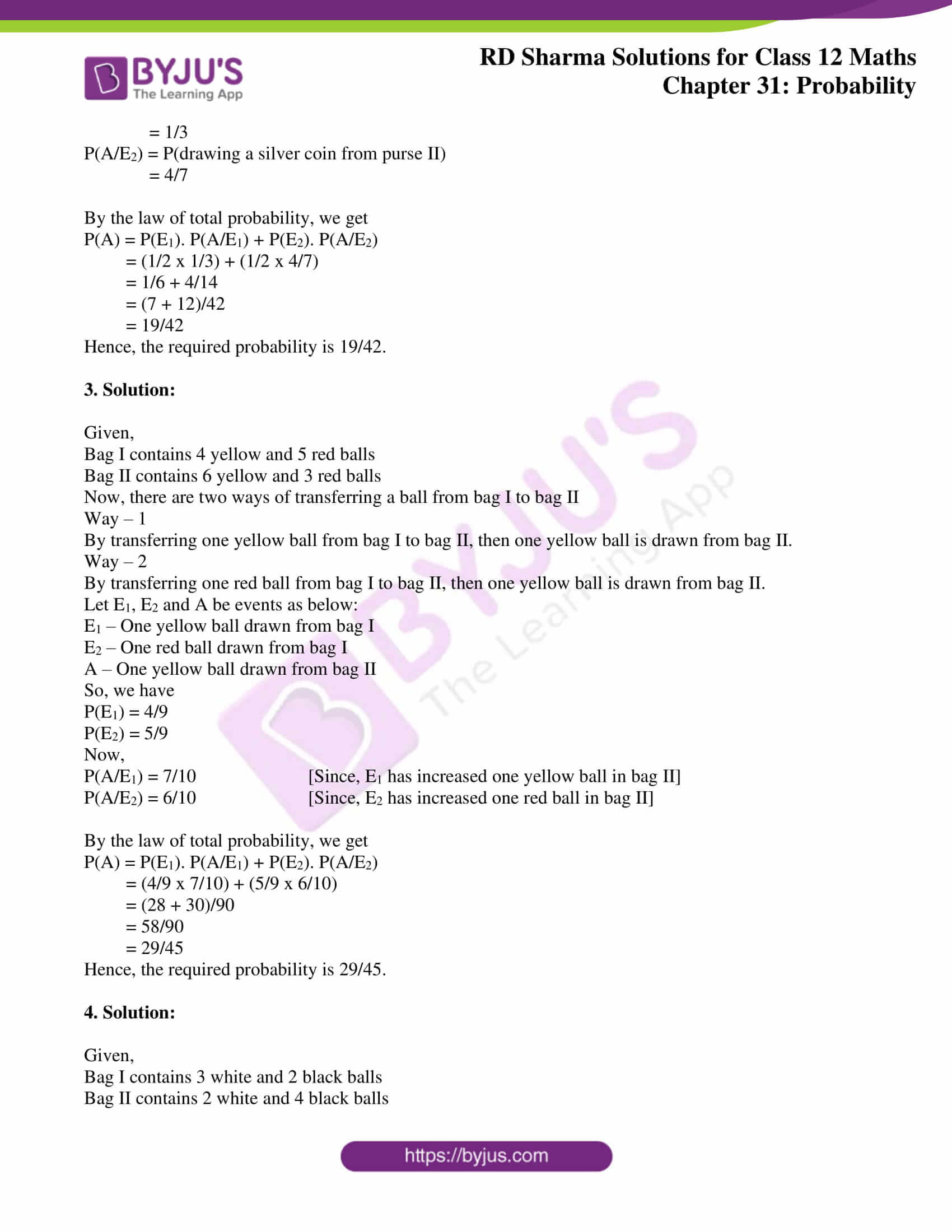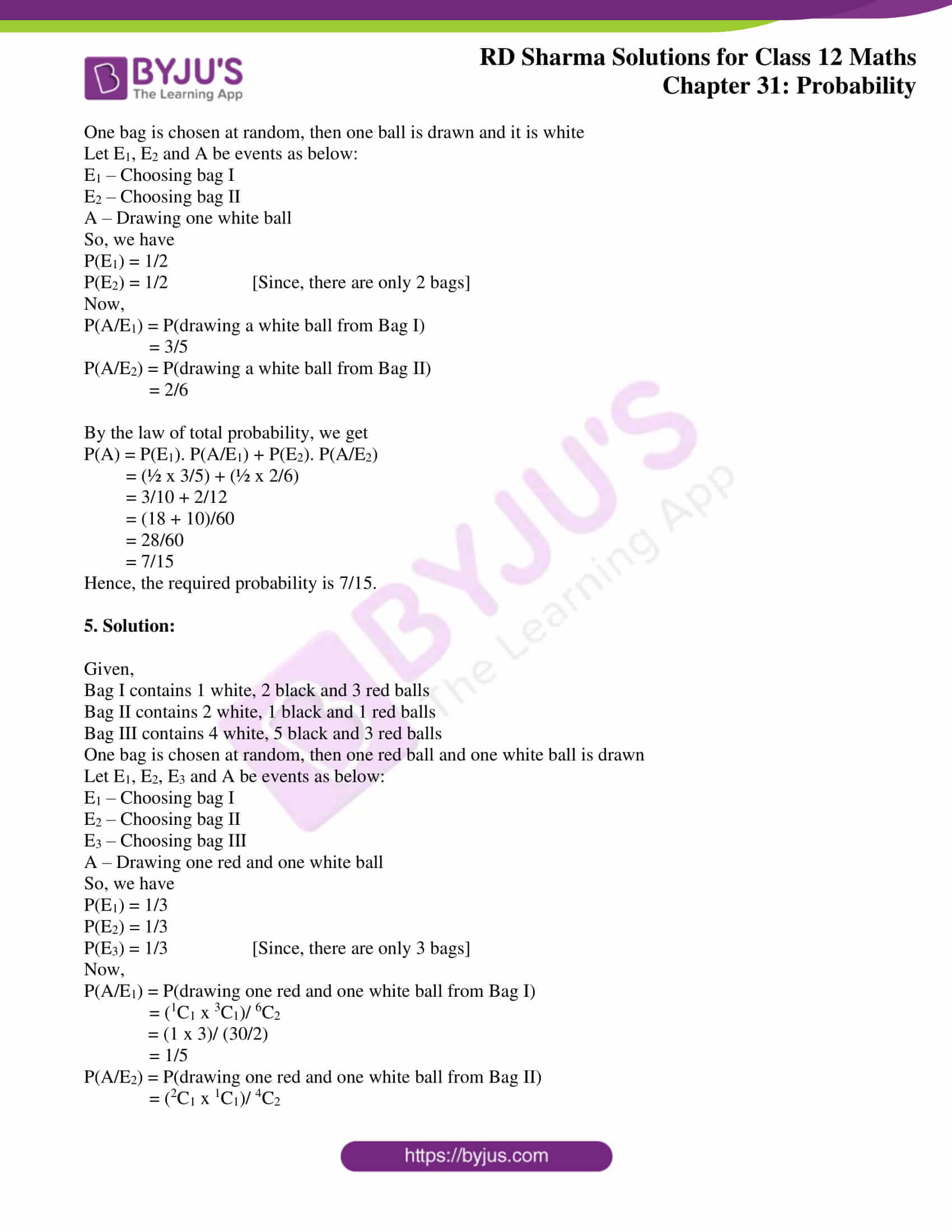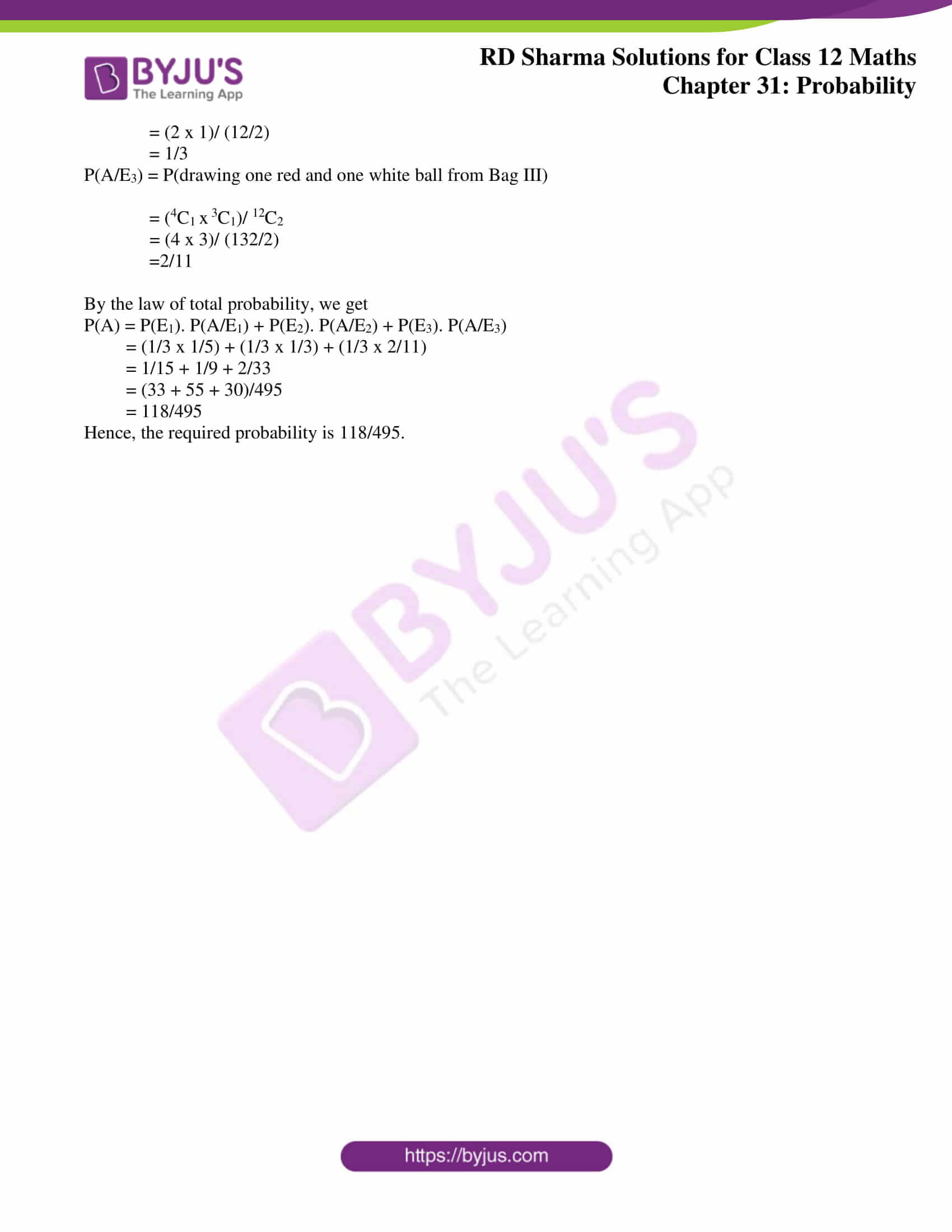# RD Sharma Solutions Class 12 Probability Exercise 31.6

RD Sharma Solutions for Class 12 Maths Chapter 31 Probability Exercise 31.6 are available here. The subject matter experts at BYJU’S have broken down the solutions of difficult problems into simple steps so that they can be easily inferred by all students with precision. Regular usage of this resource will improve problem-solving skills, which is vital from the examination point of view. Students can now start practising these solutions offline by downloading the PDF of RD Sharma Solutions for Class 12 from the link attached below.

## Download PDF of RD Sharma Solutions for Class 12 Maths Chapter 31 Exercise 6### Access RD Sharma Solutions for Class 12 Maths Chapter 31 Exercise 6

Exercise 31.6

1. Solution:

Given,

Bag A contains 5 white and 6 black balls

Bag B contains 4 white and 3 black balls

Now, there are two ways of transferring a ball from bag A to bag B

Way – 1

By transferring one white ball from bag A to bag B, then drawing one black ball from bag B.

Way – 2

By transferring one black ball from bag A to bag B, then drawing one black ball from bag B.

Let E1, E2 and A be events as below:

E1 – One white ball drawn from bag A

E2 – One black ball drawn from bag A

A – One black ball drawn from bag B

So, we have

P(E1) = 5/11

P(E2) = 6/11

Now,

P(A/E1) = 3/8 [Since, E1 has increased one white ball in bag B]

P(A/E2) = 4/8 [Since, E2 has increased one black ball in bag B]

By the law of total probability, we get

P(A) = P(E1). P(A/E1) + P(E2). P(A/E2)

= (5/11 x 3/8) + (6/11 x 4/8)

= 15/88 + 24/88

= 39/88

Hence, the required probability is 39/88.

2. Solution:

Given,

Purse (I) contains 2 silver and 4 copper coins

Purse (II) contains 4 silver and 3 copper coins

Now, one coin is drawn from one of the two purse and it is silver

Let E1, E2 and A be events as below:

E1 – selecting purse I

E2 – selecting purse II

A – drawing a silver coin

So, we have

P(E1) = 1/2

P(E2) = 1/2 [As there are only 2 purses]

Now,

P(A/E1) = P(drawing a silver from purse I)

= 2/6

= 1/3

P(A/E2) = P(drawing a silver coin from purse II)

= 4/7

By the law of total probability, we get

P(A) = P(E1). P(A/E1) + P(E2). P(A/E2)

= (1/2 x 1/3) + (1/2 x 4/7)

= 1/6 + 4/14

= (7 + 12)/42

= 19/42

Hence, the required probability is 19/42.

3. Solution:

Given,

Bag I contains 4 yellow and 5 red balls

Bag II contains 6 yellow and 3 red balls

Now, there are two ways of transferring a ball from bag I to bag II

Way – 1

By transferring one yellow ball from bag I to bag II, then one yellow ball is drawn from bag II.

Way – 2

By transferring one red ball from bag I to bag II, then one yellow ball is drawn from bag II.

Let E1, E2 and A be events as below:

E1 – One yellow ball drawn from bag I

E2 – One red ball drawn from bag I

A – One yellow ball drawn from bag II

So, we have

P(E1) = 4/9

P(E2) = 5/9

Now,

P(A/E1) = 7/10 [Since, E1 has increased one yellow ball in bag II]

P(A/E2) = 6/10 [Since, E2 has increased one red ball in bag II]

By the law of total probability, we get

P(A) = P(E1). P(A/E1) + P(E2). P(A/E2)

= (4/9 x 7/10) + (5/9 x 6/10)

= (28 + 30)/90

= 58/90

= 29/45

Hence, the required probability is 29/45.

4. Solution:

Given,

Bag I contains 3 white and 2 black balls

Bag II contains 2 white and 4 black balls

One bag is chosen at random, then one ball is drawn and it is white

Let E1, E2 and A be events as below:

E1 – Choosing bag I

E2 – Choosing bag II

A – Drawing one white ball

So, we have

P(E1) = 1/2

P(E2) = 1/2 [Since, there are only 2 bags]

Now,

P(A/E1) = P(drawing a white ball from Bag I)

= 3/5

P(A/E2) = P(drawing a white ball from Bag II)

= 2/6

By the law of total probability, we get

P(A) = P(E1). P(A/E1) + P(E2). P(A/E2)

= (½ x 3/5) + (½ x 2/6)

= 3/10 + 2/12

= (18 + 10)/60

= 28/60

= 7/15

Hence, the required probability is 7/15.

5. Solution:

Given,

Bag I contains 1 white, 2 black and 3 red balls

Bag II contains 2 white, 1 black and 1 red balls

Bag III contains 4 white, 5 black and 3 red balls

One bag is chosen at random, then one red ball and one white ball is drawn

Let E1, E2, E3 and A be events as below:

E1 – Choosing bag I

E2 – Choosing bag II

E3 – Choosing bag III

A – Drawing one red and one white ball

So, we have

P(E1) = 1/3

P(E2) = 1/3

P(E3) = 1/3 [Since, there are only 3 bags]

Now,

P(A/E1) = P(drawing one red and one white ball from Bag I)

= (1C1 x 3C1)/ 6C2

= (1 x 3)/ (30/2)

= 1/5

P(A/E2) = P(drawing one red and one white ball from Bag II)

= (2C1 x 1C1)/ 4C2

= (2 x 1)/ (12/2)

= 1/3

P(A/E3) = P(drawing one red and one white ball from Bag III)

= (4C1 x 3C1)/ 12C2

= (4 x 3)/ (132/2)

=2/11

By the law of total probability, we get

P(A) = P(E1). P(A/E1) + P(E2). P(A/E2) + P(E3). P(A/E3)

= (1/3 x 1/5) + (1/3 x 1/3) + (1/3 x 2/11)

= 1/15 + 1/9 + 2/33

= (33 + 55 + 30)/495

= 118/495

Hence, the required probability is 118/495.